JEE  >  TEST  >  JEE Main Mock - 5 (Previous Pattern)

# JEE Main Mock - 5 (Previous Pattern)

Test Description

## 90 Questions MCQ Test TEST | JEE Main Mock - 5 (Previous Pattern)

JEE Main Mock - 5 (Previous Pattern) for JEE 2022 is part of TEST preparation. The JEE Main Mock - 5 (Previous Pattern) questions and answers have been prepared according to the JEE exam syllabus.The JEE Main Mock - 5 (Previous Pattern) MCQs are made for JEE 2022 Exam. Find important definitions, questions, notes, meanings, examples, exercises, MCQs and online tests for JEE Main Mock - 5 (Previous Pattern) below.
Solutions of JEE Main Mock - 5 (Previous Pattern) questions in English are available as part of our TEST for JEE & JEE Main Mock - 5 (Previous Pattern) solutions in Hindi for TEST course. Download more important topics, notes, lectures and mock test series for JEE Exam by signing up for free. Attempt JEE Main Mock - 5 (Previous Pattern) | 90 questions in 180 minutes | Mock test for JEE preparation | Free important questions MCQ to study TEST for JEE Exam | Download free PDF with solutions
 1 Crore+ students have signed up on EduRev. Have you?
JEE Main Mock - 5 (Previous Pattern) - Question 1

### The innermost orbit of the hydrogen atom has a diameter 1.06 Å. The diameter of tenth orbit is

Detailed Solution for JEE Main Mock - 5 (Previous Pattern) - Question 1 Radius of an atom is directly proportional to n^2 and
radius of nth orbit = Radius of first orbit multiplied by n^2
so that will give you
diameter of n = 10 orbit
will be

1.06x (10)^2= 106 Å
JEE Main Mock - 5 (Previous Pattern) - Question 2

### A resistance of 40 ohm and an inductance of 95.5 millihenry are connected in series in a 50 cycles/second A.C. circuit. The impedance of this combination is very nearly

Detailed Solution for JEE Main Mock - 5 (Previous Pattern) - Question 2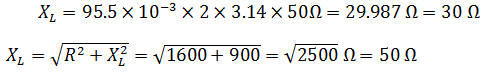JEE Main Mock - 5 (Previous Pattern) - Question 3

### An object of mass 10 kg is whirled round a horizontal circle of radius 4 m by a revolving string inclined 30º to the vertical. If the uniform speed of the object is 5 ms⁻1, the tension in the string is

JEE Main Mock - 5 (Previous Pattern) - Question 4

A coil of 40 H inductance is connected in series with a resistance of 8 Ω and the combination is joined to the terminals of a 2 V battery. The time-constant of the circuit is

Detailed Solution for JEE Main Mock - 5 (Previous Pattern) - Question 4
In case of an inductive resistive circuit, the time constant is given as L(eq)/R(eq).
So, in the given case
L(eq)=40H
R(eq)=8 ohm
Thus, time cons.=40/8=5s
JEE Main Mock - 5 (Previous Pattern) - Question 5

For the production of X-rays, the target should be made of

JEE Main Mock - 5 (Previous Pattern) - Question 6

The mass of photon at rest is

JEE Main Mock - 5 (Previous Pattern) - Question 7

An electric dipole is placed along the X-axis at the origin O. A point P is at a distance of 20 cm from this origin such that OP makes an angle π/3 with the X axis. If electric field at P makes an angle θ with X-axis, the value of θ is

JEE Main Mock - 5 (Previous Pattern) - Question 8

Two charged conducting spheres of radii a and b are connected to each other by a wire. The ratio of electric fields at the surface of two spheres is

Detailed Solution for JEE Main Mock - 5 (Previous Pattern) - Question 8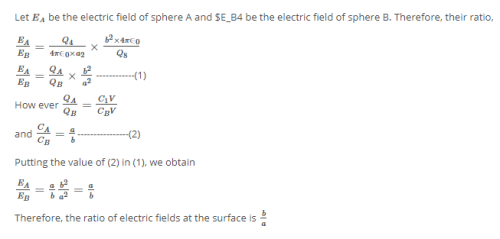JEE Main Mock - 5 (Previous Pattern) - Question 9

A lift is moving upward with increasing speed with acceleration 'a'. The apparent weight will be

JEE Main Mock - 5 (Previous Pattern) - Question 10

If the coefficient of friction between the tyres and the road is 0.4, then maximum speed of a car on a road-turn of radius 30 m, will be

Detailed Solution for JEE Main Mock - 5 (Previous Pattern) - Question 10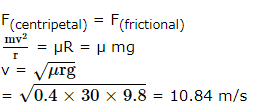JEE Main Mock - 5 (Previous Pattern) - Question 11

Two NOT gates are connected at the two inputs of a NAND gate. This combination will behave like

JEE Main Mock - 5 (Previous Pattern) - Question 12

A long solenoid has a radius a and number of turns per unit length is n. If it carries a current I, then the magnetic field on its axis is directly propotional to

JEE Main Mock - 5 (Previous Pattern) - Question 13

A horizontal pipe line carries water in a streamline flow. At a point along the pipe where cross-sectional area is 10 cm2, the velocity of water is 1 m-s⁻1 and pressure is 2000 Pa. The pressure of water, at another point where cross-sectional area is 5 cm2, is (Density of water = 1000 kg-m⁻3)

JEE Main Mock - 5 (Previous Pattern) - Question 14

A particle has an initial velocity of 3 i ‸ + 4 j ‸ and an acceleration of 0.4 i ‸ + 0.3 j ‸ . Its speed after 10s is-

JEE Main Mock - 5 (Previous Pattern) - Question 15

If in a nuclear reactor using U235 as fuel, the power output is 4.8 mega watts, the number of fissions per second is:
(energy released per fission of U235 = 200 MeV; 1eV = 1.6 x 10 − 19 J)

JEE Main Mock - 5 (Previous Pattern) - Question 16

In the following question, a Statement of Assertion (A) is given followed by a corresponding Reason (R) just below it. Read the Statements carefully and mark the correct answer-
Assertion(A): Air quickly leaking out of a balloon becomes cooler.
Reason(R): The leaking air undergoes adiabatic expansion. Reason(R): The leaking air undergoes adiabatic expansion.

JEE Main Mock - 5 (Previous Pattern) - Question 17

In the following question, a Statement of Assertion (A) is given followed by a corresponding Reason (R) just below it. Read the Statements carefully and mark the correct answer-
Assertion(A):If the string of a descending lift is broken, then it will fall down like a free body.
Reason(R):The acceleration of a free body is always 'g'.

JEE Main Mock - 5 (Previous Pattern) - Question 18

A circular disc is rotating with angular velocity ω. A man sitting at the edge walks towards the centre of disc, then the angular velocity ω

JEE Main Mock - 5 (Previous Pattern) - Question 19

During an adiabatic process, the pressure of a gas is proportional to the cube of its adiabatic temperature. The value of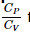for that gas is

JEE Main Mock - 5 (Previous Pattern) - Question 20

SI unit of magnetic dipole moment is

JEE Main Mock - 5 (Previous Pattern) - Question 21

The maximum number of possible interference maxima for slit-separation equal to twice the wavelength in Young's double-slit experiment, is

Detailed Solution for JEE Main Mock - 5 (Previous Pattern) - Question 21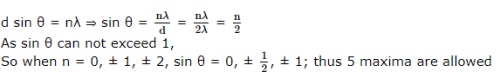JEE Main Mock - 5 (Previous Pattern) - Question 22

Two identical light waves, propagating in the same direction, have a phase difference δ . After they superpose the intensity of the resulting wave will be proportional to

JEE Main Mock - 5 (Previous Pattern) - Question 23

When exposed to sunlight, thin films of oil on water often exhibit brilliant colours due to the phenomenon of

JEE Main Mock - 5 (Previous Pattern) - Question 24

The intensity of a harmonic wave

JEE Main Mock - 5 (Previous Pattern) - Question 25

The transition from the state n = 4 to n = 3 in hydrogen like atom results in ultraviolet radiation. Infrared radiation will be obtained in the transition from-

JEE Main Mock - 5 (Previous Pattern) - Question 26

A capacitor of capacity C₁ is charged by connecting it across a battery of e.m.f. V₀. The battery is then removed and the capacitor is connected in parallel with an uncharged capacitor of capacity C₂. The potential difference across this combination is

JEE Main Mock - 5 (Previous Pattern) - Question 27

If a particle of mass m is projected from the lowest point of smooth vertical circle of radius 'A' with velocity 5 a g the pressure at its lowest point will be—

JEE Main Mock - 5 (Previous Pattern) - Question 28

A series combination of two resistors 1 Ω each is connected to a 12 V battery of internal resistance 0.4Ω. The current flowing through it is

JEE Main Mock - 5 (Previous Pattern) - Question 29

The figure to the right shows an edge-on view of three extremely large, parallel insulating sheets positively charged with uniform and identical surface charge densities. In which of the regions shown is the electric field a maximum?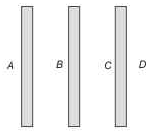JEE Main Mock - 5 (Previous Pattern) - Question 30

A straight wire of diameter 0.5 mm carrying a current of 1 A is replaced by another wire of 1 mm diameter carrying the same current. The strength of magnetic field far away is

JEE Main Mock - 5 (Previous Pattern) - Question 31

Ethanol when reacted with PCl₅ gives A, POCl₃ and HCl. A reacts with silver nitrite to form B (major product) and AgCl. A and B respectively are :

JEE Main Mock - 5 (Previous Pattern) - Question 32

Benzyl alcohol is obtained from benzaldehyde by

JEE Main Mock - 5 (Previous Pattern) - Question 33

The enzyme pepsin hydrolyses

JEE Main Mock - 5 (Previous Pattern) - Question 34

For a reaction, 2A + B → Products, the active mass of B is kept constant and that of A is doubled. The rate of reaction will then

JEE Main Mock - 5 (Previous Pattern) - Question 35

A liquid is in equilibrium with its vapour at its boiling point. The molecules in these two phases will have equal

JEE Main Mock - 5 (Previous Pattern) - Question 36

The densities of graphite and diamond at 298 K are 2.25 and 3.31 g-cm-3 respectively. If the standard free energy difference (ΔGº)is equal to 1895 J-mol-1, then pressure at which graphite will be transformed into diamond at 298 K is

JEE Main Mock - 5 (Previous Pattern) - Question 37

Which of the following elements has the lowest ionisation potential

Detailed Solution for JEE Main Mock - 5 (Previous Pattern) - Question 37

The ionisation potential increases from O to F to Ne, because of increasing nuclear charge. The ionisation potential is highest for Ne because of its stable (ns2 np6) electronic configuration. But the ionisation potential of O is lower than N although the nuclear charge of O is higher. This is due to the extra-stability of exactly half-filled 2p-orbitals of N.

JEE Main Mock - 5 (Previous Pattern) - Question 38

The most stable complex among the following is

JEE Main Mock - 5 (Previous Pattern) - Question 39

In the diazotisation of aniline the excess of hydrochloric acid is used primarily with sodium nitrite because it

JEE Main Mock - 5 (Previous Pattern) - Question 40

The unit ohm⁻1 is used for

JEE Main Mock - 5 (Previous Pattern) - Question 41

The pair of compounds, in which both the metals are in their highest possible oxidation state, is

JEE Main Mock - 5 (Previous Pattern) - Question 42

An aqueous solution of CuSO₄ is

JEE Main Mock - 5 (Previous Pattern) - Question 43

The aqueous solution of which of the following salt has the lowest pH value?

JEE Main Mock - 5 (Previous Pattern) - Question 44

Why only As⁺³ gets precipitated as As₂S₃ and not Zn⁺² as ZnS when H₂S is passed through an acidic solution containing As⁺³ and Zn⁺²?

JEE Main Mock - 5 (Previous Pattern) - Question 45

What is the conjugate base of OH⁻?

JEE Main Mock - 5 (Previous Pattern) - Question 46

Which one of the following compounds will be most readily attacked by an electrophile?

JEE Main Mock - 5 (Previous Pattern) - Question 47

Following carbocation changes to more stable carbocation :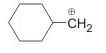JEE Main Mock - 5 (Previous Pattern) - Question 48

An example of natural biopolymer is

JEE Main Mock - 5 (Previous Pattern) - Question 49

Ethane is obtained from ethylene by which of the following methods?

JEE Main Mock - 5 (Previous Pattern) - Question 50

A face-centred cubic element has a edge length of 400 pm. What is its density? At. weight of element= 60)

JEE Main Mock - 5 (Previous Pattern) - Question 51

Which of the following does not represent a type of crystal system

JEE Main Mock - 5 (Previous Pattern) - Question 52

2N-HCl will have the same molar concentration is

JEE Main Mock - 5 (Previous Pattern) - Question 53

An azeotropic mixture of two liquids boils at a lower temperature than either of these when

JEE Main Mock - 5 (Previous Pattern) - Question 54

What is formed when calcium carbide reacts with heavy water?

JEE Main Mock - 5 (Previous Pattern) - Question 55

For n = 5, total number of electrons will be ___Options

JEE Main Mock - 5 (Previous Pattern) - Question 56

If Z-axis is te molecular axis, which of the following combination is not allowed for the formation of MO?Options

JEE Main Mock - 5 (Previous Pattern) - Question 57

Which of the following statements is true ?Options

JEE Main Mock - 5 (Previous Pattern) - Question 58

For a second order reaction, the time required for half change is _Options

JEE Main Mock - 5 (Previous Pattern) - Question 59

Which one of the following high-spin complexes has the largest CFSE (Crystal field stabilization energy)?Options

Detailed Solution for JEE Main Mock - 5 (Previous Pattern) - Question 59

Cation with higher oxidation state has a larger value of CFSE and CFSE decrease with the increase of the number of d-electrons.

JEE Main Mock - 5 (Previous Pattern) - Question 60

In a Lassaigne's test for sulphur in the organic compound with sodium nitroprusside solution the purple colour formed is due toOptions

JEE Main Mock - 5 (Previous Pattern) - Question 61

If (a2)+16(b2)+49(c2)-4ab-28bc-7ac=0, then a,b,c are in:

JEE Main Mock - 5 (Previous Pattern) - Question 62

The digit in the unit place of the number 2009!+378862009!+37886 is

JEE Main Mock - 5 (Previous Pattern) - Question 63

The volume of the solid obtained by rotating the ellipse (x²/a²) + (y²/b²) = 1 about axis of x isOptions

JEE Main Mock - 5 (Previous Pattern) - Question 64

Circles x²+y²-2x-4y and x²+y²-8y-4=0Options

JEE Main Mock - 5 (Previous Pattern) - Question 65

The centre and radius of the circle with the segment of the line x+y=1 cut of by the coordinate axes as diameter areOptions

JEE Main Mock - 5 (Previous Pattern) - Question 66

If ω is non-real cube root of unity then [(1 + 2ω + 3ω²) /(2 + 3ω + ω²)] + [(2 + 3ω + 3ω²)/(3 + 3ω + 2ω²)] is equal toOptions

JEE Main Mock - 5 (Previous Pattern) - Question 67

The argument of the complex number 13−5i4−9i13−5i4−9i isOptions

JEE Main Mock - 5 (Previous Pattern) - Question 68

If y (t) is solution of [(t +(dy∕dt)] - ty = 1, y (0) = -1. At t = 1 the solution is

JEE Main Mock - 5 (Previous Pattern) - Question 69

If f(x)=cosx, 0≤x≤(π/2), the value of 'c' for which f(x) satisfies Mean Value theorem isOptions

JEE Main Mock - 5 (Previous Pattern) - Question 70

If g(x) = x² + x - 2 and 1/2 (gof) (x) = 2x² - 5x + 2, then f(x) is equal toOptions

JEE Main Mock - 5 (Previous Pattern) - Question 71

Which of the following is not a statement in logic ?
1. Earth is planet .
2. Plants are living object .
3. √−3−3 is rational number .
4. x2 - 5x + 6 < 0, where x ∈−R∈−R

JEE Main Mock - 5 (Previous Pattern) - Question 72

In the following question, a Statement of Assertion (A) is given followed by a corresponding Reason (R) just below it. Read the Statements carefully and mark the correct answer-
Assertion(A): The value of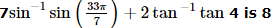Reason(R) :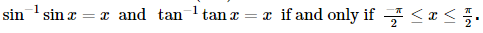JEE Main Mock - 5 (Previous Pattern) - Question 73

In the following question, a Statement of Assertion (A) is given followed by a corresponding Reason (R) just below it. Read the Statements carefully and mark the correct answer-
Assertion(A): For x = r cos θ, y = r sin θ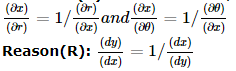JEE Main Mock - 5 (Previous Pattern) - Question 74

The number 4.4 × 106 ohms expressed using a metric prefix is

JEE Main Mock - 5 (Previous Pattern) - Question 75

Equation of the tangent at (-4,-4) on x²=-4y isOptions

JEE Main Mock - 5 (Previous Pattern) - Question 76

The number of diagonals that can be drawn by joining the vertices of a heptagon is :Options

JEE Main Mock - 5 (Previous Pattern) - Question 77

The probability of sure event isOptions

JEE Main Mock - 5 (Previous Pattern) - Question 78

If x and y are related as y - 4x = 3, then the nature of correlation between x and y isOptions

JEE Main Mock - 5 (Previous Pattern) - Question 79

The foot of perpendicular from (2,4) on the line x+y=1 isOptions

JEE Main Mock - 5 (Previous Pattern) - Question 80

The equation of the normal to the curve y=sinx at (π,0) isOptions

JEE Main Mock - 5 (Previous Pattern) - Question 81

Which of the following lines passes through the centre of the sphere x² + y² + z² + 4x - 4z = 0Options

JEE Main Mock - 5 (Previous Pattern) - Question 82

The one root of the equation cosx-x+(1/2)=0 lies in the intervalOptions

JEE Main Mock - 5 (Previous Pattern) - Question 83

If the position vectors of A and B are i+3j-k and 3i-j-3k respectively, then the position vector of mid point of AB isOptions

JEE Main Mock - 5 (Previous Pattern) - Question 84

If a = i + 2 j + 3k, b = - i + 2 j + k and c = 3 i + j and a + tb is perpendicular to c, the value of t is

Detailed Solution for JEE Main Mock - 5 (Previous Pattern) - Question 84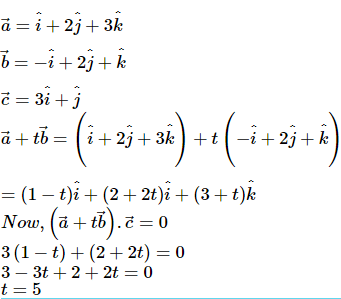JEE Main Mock - 5 (Previous Pattern) - Question 85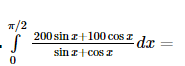JEE Main Mock - 5 (Previous Pattern) - Question 86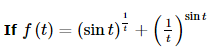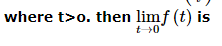JEE Main Mock - 5 (Previous Pattern) - Question 87

In the following question, a Statement of Assertion (A) is given followed by a corresponding Reason (R) just below it. Read the Statements carefully and mark the correct answer-
Assertion(A):The inverse of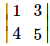does not exist.
Reason(R): The matrix is non-singular.

JEE Main Mock - 5 (Previous Pattern) - Question 88

If A is a singular matrix, then A adj (A)

JEE Main Mock - 5 (Previous Pattern) - Question 89

The minimum distance from the point (4, 2) to the parabola y2 = 8x isOptions

JEE Main Mock - 5 (Previous Pattern) - Question 90

Let f(x) =ax²+bx+c,a≠0 and triangle = b²-4ac.If α+β, α²+β² and α³+β³ are in G.P., then :Options

## TEST

15 tests
 Use Code STAYHOME200 and get INR 200 additional OFF Use Coupon Code
Information about JEE Main Mock - 5 (Previous Pattern) Page
In this test you can find the Exam questions for JEE Main Mock - 5 (Previous Pattern) solved & explained in the simplest way possible. Besides giving Questions and answers for JEE Main Mock - 5 (Previous Pattern), EduRev gives you an ample number of Online tests for practice

15 tests